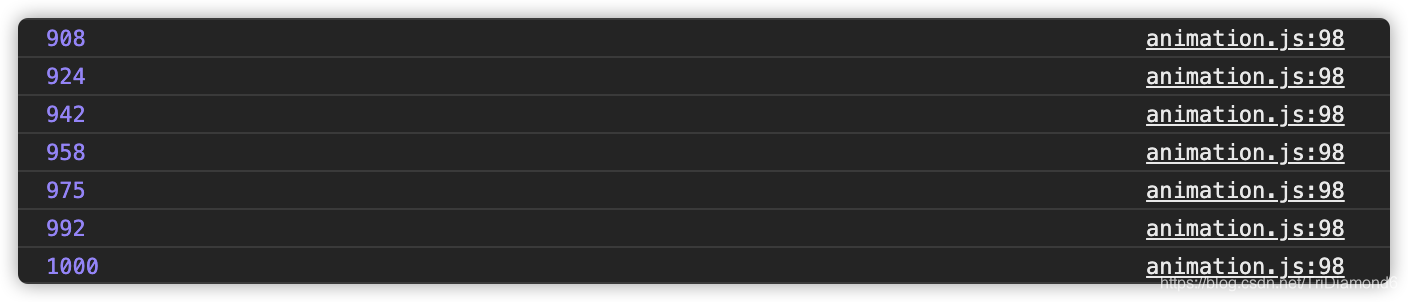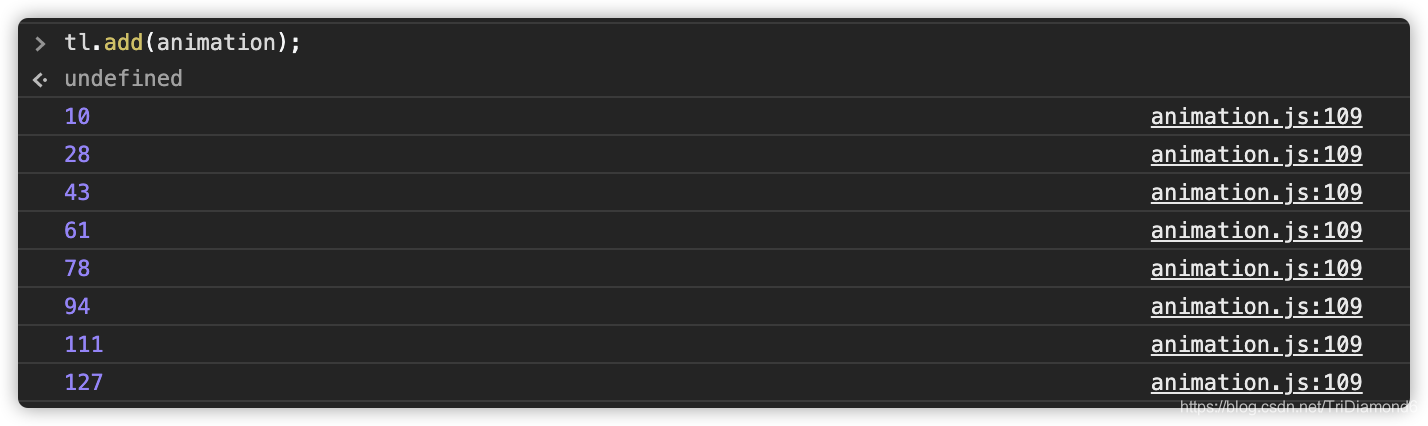# 使用JavaScript 实现时间轴与动画效果的示例代码(前端组件化)

2021-04-08 04:06 88 查看

## 代码整理

carousel.js 中只需要 import `Component` 即可，然后给我们的 Carousel 类加上 export。代码结构如下：

```import { Component } from './framework.js';

export class Carousel extends Component {/** Carousel 里面的代码 */}```

```import { Component, createElement } from './framework.js';
import { Carousel } from './carousel.js';

let gallery = [
'https://source.unsplash.com/Y8lCoTRgHPE/1600x900',
'https://source.unsplash.com/v7daTKlZzaw/1600x900',
'https://source.unsplash.com/DlkF4-dbCOU/1600x900',
'https://source.unsplash.com/8SQ6xjkxkCo/1600x900',
];

let a = <Carousel src={gallery} />;

// document.body.appendChild(a);
a.mountTo(document.body);```

## 实现“帧”的方法

### 1. setInterval

`setInterval(() => {/** 一帧要发生的事情 */}, 16)`

### 2. setTimeout

```let tick = () => {
/** 我们的逻辑/事件 */
setTimout(tick, 16);
}```

### 3. requestAnimationFrame

```let tick = () => {
/** 我们的逻辑/事件 */
setTimout(tick, 16);
}```

“为什么不用 setInterval 呢”？因为 setInterval 比较不可控，浏览器到底会不会按照我们设置的 16 毫秒去执行呢？这个就不好说了。

```let tick = () => {
let handler = requestAnimationFrame(tick);
cancelAnimationFrame(handler);
}```

## 实现 start 函数

```const TICK = Symbol('tick');
const TICK_HANDLER = Symbol('tick-handler');

export class Timeline {
constructor() {
this[TICK] = () => {
console.log('tick');
requestAnimationFrame(this[TICK]);
};
}
start() {
this[TICK]();
}
pause() {}
resume() {}
reset() {}
}```

```import { Timeline } from './animation.js';

let tl = new Timeline();

tl.start();```Build 一下我们的代码，然后在浏览器运行，这时候就可以看到在 console 中，我们的 tick 是正常在运行了。这说明我们 Timeline 目前的逻辑是写对了。

## 实现 Animation 类

```export class Animation {
constructor() {}
}```

• `object`：被赋予动画的元素对象
• `property`：被赋予动画变动的属性
• `startValue`：动画起始值
• `endValue`：动画终止值
• `duration`：动画时长
• `timingFunction`：动画与时间的曲线

```export class Animation {
constructor(object, property, startValue, endValue, duration, timingFunction) {
this.object = object;
this.property = property;
this.startValue = startValue;
this.endValue = endValue;
this.duration = duration;
this.timingFunction = timingFunction;
}
}```

`变化值 = 变化区间值(range) * 时间(time) / 动画时长(duration)`

```run(time) {
let range = this.endValue - this.startValue;
this.object[this.property] = this.startValue + (range * time) / this.duration;
}```

`const ANIMATIONS = Symbol('animations');`

```constructor() {
this[ANIMATIONS] = new Set();
}```

```constructor() {
this[ANIMATIONS] = new Set();
}```

```const TICK = Symbol('tick');
const TICK_HANDLER = Symbol('tick-handler');const ANIMATIONS = Symbol('animations');

export class Timeline {constructor() {
this[ANIMATIONS] = new Set();
}
start() {
let startTime = Date.now();
this[TICK] = () => {
let t = Date.now() - startTime;
for (let animation of this[ANIMATIONS]) {
animation.run(t);
}
requestAnimationFrame(this[TICK]);
};
this[TICK]();
}
pause() {}
resume() {}
reset() {}
add(animation) {
this[ANIMATIONS].add(animation);
}
}

export class Animation {
constructor(object, property, startValue, endValue, duration, timingFunction) {
this.object = object;
this.property = property;
this.startValue = startValue;
this.endValue = endValue;
this.duration = duration;
this.timingFunction = timingFunction;
}

run(time) {
console.log(time);
let range = this.endValue - this.startValue;
this.object[this.property] = this.startValue + (range * time) / this.duration;
}
}```

```import { Component, createElement } from './framework.js';
import { Carousel } from './carousel.js';
import { Timeline, Animation } from './animation.js';

let gallery = [
'https://source.unsplash.com/Y8lCoTRgHPE/1600x900',
'https://source.unsplash.com/v7daTKlZzaw/1600x900',
'https://source.unsplash.com/DlkF4-dbCOU/1600x900',
'https://source.unsplash.com/8SQ6xjkxkCo/1600x900',
];

let a = <Carousel src={gallery} />;

// document.body.appendChild(a);
a.mountTo(document.body);

let tl = new Timeline();
// tl.add(new Animation({}, 'property', 0, 100, 1000, null));

tl.start();``````export class Timeline {constructor() {
this[ANIMATIONS] = new Set();
}
start() {
let startTime = Date.now();
this[TICK] = () => {
let t = Date.now() - startTime;
for (let animation of this[ANIMATIONS]) {
if (t > animation.duration) {
this[ANIMATIONS].delete(animation);
}
animation.run(t);
}
requestAnimationFrame(this[TICK]);
};
this[TICK]();
}
pause() {}
resume() {}
reset() {}
add(animation) {
this[ANIMATIONS].add(animation);
}
}```

```tl.add(
new Animation(
{
set a(a) {
console.log(a);
},
},
'property',
0,
100,
1000,
null
)
);``````start() {
let startTime = Date.now();
this[TICK] = () => {
let t = Date.now() - startTime;
for (let animation of this[ANIMATIONS]) {
let t0 = t;
if (t > animation.duration) {
this[ANIMATIONS].delete(animation);
t0 = animation.duration;
}
animation.run(t0);
}
requestAnimationFrame(this[TICK]);
};
this[TICK]();
}
pause() {}
resume() {}
reset() {}
add(animation) {
this[ANIMATIONS].add(animation);
}
}```## 添加 Delay 属性支持

```export class Animation {
constructor(object, property, startValue, endValue, duration, delay, timingFunction) {
this.object = object;
this.property = property;
this.startValue = startValue;
this.endValue = endValue;
this.duration = duration;
this.timingFunction = timingFunction;
this.delay = delay;
}

run(time) {
console.log(time);
let range = this.endValue - this.startValue;
this.object[this.property] = this.startValue + (range * time) / this.duration;
}
}```

```export class Animation {
constructor(object, property, startValue, endValue, duration, delay, timingFunction) {
this.object = object;
this.property = property;
this.startValue = startValue;
this.endValue = endValue;
this.duration = duration;
this.timingFunction = timingFunction;
this.delay = delay;
}

run(time) {
console.log(time);
let range = this.endValue - this.startValue;
this.object[this.property] = this.startValue + (range * time) / this.duration;
}
}```

```add(animation, startTime) {
if (arguments.length < 2) startTime = Date.now();
this[ANIMATIONS].add(animation);
this[START_TIMES].set(animation, startTime);
}```

• 第一种情况： 如果我们动画的开始时间是小于，Timeline 的开始时间的，那么我们当前动画的时间进度就是 `当前时间 - Timeline 开始时间`
• 第二种情况： 动画的开始时间大于 Timeline 的开始时间，那么当前动画的时间进度就是 `当前时间 - 动画的开始时间`

```start() {
let startTime = Date.now();
this[TICK] = () => {
let now = Date.now();
for (let animation of this[ANIMATIONS]) {
let t;

if (this[START_TIMES].get(animation) < startTime) {
t = now - startTime;
} else {
t = now - this[START_TIMES].get(animation);
}

if (t > animation.duration) {
this[ANIMATIONS].delete(animation);
t = animation.duration;
}
animation.run(t);
}
requestAnimationFrame(this[TICK]);
};
this[TICK]();
}```

```start() {
let startTime = Date.now();
this[TICK] = () => {
let now = Date.now();
for (let animation of this[ANIMATIONS]) {
let t;

if (this[START_TIMES].get(animation) < startTime) {
t = now - startTime;
} else {
t = now - this[START_TIMES].get(animation);
}

if (t > animation.duration) {
this[ANIMATIONS].delete(animation);
t = animation.duration;
}
animation.run(t);
}
requestAnimationFrame(this[TICK]);
};
this[TICK]();
}```

`tl.add(animation);````if (this[START_TIMES].get(animation) < startTime) {
t = now - startTime - animation.delay;
} else {
t = now - this[START_TIMES].get(animation) - animation.delay;
}```

`if (t > 0) animation.run(t);`

## 实现 Pause

```start() {
let startTime = Date.now();
this[TICK] = () => {
let now = Date.now();
for (let animation of this[ANIMATIONS]) {
let t;
if (this[START_TIMES].get(animation) < startTime) {
t = now - startTime - animation.delay;
} else {
t = now - this[START_TIMES].get(animation) - animation.delay;
}

if (t > animation.duration) {
this[ANIMATIONS].delete(animation);
t = animation.duration;
}if (t > 0) animation.run(t);
}
this[TICK_HANDLER] = requestAnimationFrame(this[TICK]);
};
this[TICK]();
}```

```pause() {
cancelAnimationFrame(this[TICK_HANDLER]);
}```

Pause（暂停） 还是比较简单的，但是 resume（重启）就比较复杂了。

## 实现 Resume

```<!-- 新建立一个 animation.html (放在 dist 文件夹里面) -->

<style>
.box {
width: 100px;
height: 100px;
background-color: aqua;
}
</style>

<body>
<div class="box"></div>
<script src="./main.js"></script>
</body>```

```// 在根目录建立一个 `animation-demo.js`
import { Timeline, Animation } from './animation.js';

let tl = new Timeline();

tl.start();tl.add(
new Animation(
{
set a(a) {
console.log(a);
},
},
'property',
0,
100,
1000,
null
)
);```

```module.exports = {
entry: './animation-demo.js',
mode: 'development',
devServer: {
contentBase: './dist',
},
module: {
rules: [
{
test: /\.js\$/,
use: {
loader: 'babel-loader',
options: {
presets: ['@babel/preset-env'],
plugins: [['@babel/plugin-transform-react-jsx', { pragma: 'createElement' }]],
},
},
},
],
},
};````<div class="box" id="el"></div>`

`v => `translate(\${\$v}px)`;`

```tl.add(
new Animation(
document.querySelector('#el').style,
'transform',
0,
100,
1000,
0,
null,
v => `translate(\${v}px)`
)
);```

```export class Animation {
constructor(
object,
property,
startValue,
endValue,
duration,
delay,
timingFunction,
template
) {
this.object = object;
this.property = property;
this.startValue = startValue;
this.endValue = endValue;
this.duration = duration;
this.timingFunction = timingFunction;
this.delay = delay;
this.template = template;
}

run(time) {
let range = this.endValue - this.startValue;
this.object[this.property] =
this.template(
this.startValue + (range * time) / this.duration
);
}
}``````tl.add(
new Animation(
document.querySelector('#el').style,
'transform',
0,
500,
2000,
0,
null,
v => `translate(\${v}px)`
)
);```

```<body><div class="box" id="el"></div>
<button id="pause-btn">Pause</button>
<script src="./main.js"></script>
</body>```

```document.querySelector('#pause-btn').addEventListener(
'click',
() => tl.pause()
);``````<!-- animation.html -->

<body><div class="box" id="el"></div>
<button id="pause-btn">Pause</button>
<button id="resume-btn">Resume</button>
<script src="./main.js"></script>
</body>```
```// animation-demo.js 中加入 resume 按钮事件绑定。

document.querySelector('#resume-btn').addEventListener(
'click',
() => tl.resume()
);```

```resume() {
this[TICK]();
}``````const PAUSE_START = Symbol('pause-start');
const PAUSE_TIME = Symbol('pause-time');```

```pause() {
this[PAUSE_START] = Date.now();
cancelAnimationFrame(this[TICK_HANDLER]);
}```

```export class Timeline {
constructor() {
this[ANIMATIONS] = new Set();
this[START_TIMES] = new Map();
}
start() {
let startTime = Date.now();
this[PAUSE_TIME] = 0;
this[TICK] = () => {
let now = Date.now();
for (let animation of this[ANIMATIONS]) {
let t;

if (this[START_TIMES].get(animation) < startTime) {
t = now - startTime - animation.delay - this[PAUSE_TIME];
} else {
t = now - this[START_TIMES].get(animation) - animation.delay - this[PAUSE_TIME];
}

if (t > animation.duration) {
this[ANIMATIONS].delete(animation);
t = animation.duration;
}if (t > 0) animation.run(t);
}
this[TICK_HANDLER] = requestAnimationFrame(this[TICK]);
};
this[TICK]();
}pause() {
this[PAUSE_START] = Date.now();
cancelAnimationFrame(this[TICK_HANDLER]);
}
resume() {
this[PAUSE_TIME] += Date.now() - this[PAUSE_START];
this[TICK]();
}
reset() {}add(animation, startTime) {
if (arguments.length < 2) startTime = Date.now();
this[ANIMATIONS].add(animation);
this[START_TIMES].set(animation, startTime);
}
}```